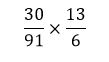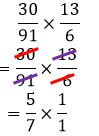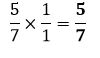# How to divide fractions

Want to find out how to divide fractions? We've provided a step-by-step guide of how to divide fractions to teach you everything you need to know.## Step 1: Determine the reciprocal of the divisor

The divisor is the fraction after the division.

## Step 2: Multiply the dividend by the reciprocal of the divisor

Note that if the dividend (fraction being divided) is a mixed fraction, make sure to change it to improper fraction.

So, for 39/40 ÷ 13/20,

## Step 3: Cancel common factors

Cancel out common factors shared between numerators and denominators to simplify the fraction.

## Examples of how to divide fractions

Q1) What would the result of 30/91 ÷ 6/13 be?

Find the reciprocal of the divisor. Reciprocal of 6/13=13/6.

Once we have the reciprocal, multiply the dividend with the divisor.Cancel out common factors shared by the numerator and the denominator.Multiply the remaining factors to simplify the product.#### Join today

The fastest way to practice

Unlock our complete testing platform and improve faster that ever.